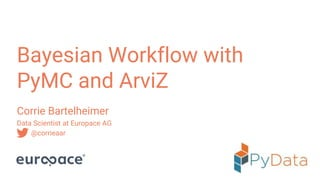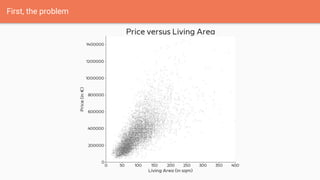Successfully reported this slideshow.

# Bayesian workflow with PyMC3 and ArviZ

2

Share×
1 of 62
1 of 62

# Bayesian workflow with PyMC3 and ArviZ

2

Share

Slides for my Talk at PyConDE/PyData Berlin 2019 https://de.pycon.org/program/pydata-rrlryg-a-bayesian-workflow-with-pymc-and-arviz-corrie-bartelheimer/

Resources:
Richard McElreath "Statistical Rethinking": https://xcelab.net/rm/statistical-rethinking/
Statistical Rethinking Port to PyMC3: https://github.com/pymc-devs/resources/tree/master/Rethinking
Prior Recommendation by Stan Team: https://github.com/stan-dev/stan/wiki/Prior-Choice-Recommendations
Michael Betancourt Case Studies: https://betanalpha.github.io/writing/
Berlin Bayesian Meetup Group: https://www.meetup.com/de-DE/BerlinBayesians/

Code and Notebook:
https://github.com/corriebar/Bayesian-Workflow-with-PyMC

Slides for my Talk at PyConDE/PyData Berlin 2019 https://de.pycon.org/program/pydata-rrlryg-a-bayesian-workflow-with-pymc-and-arviz-corrie-bartelheimer/

Resources:
Richard McElreath "Statistical Rethinking": https://xcelab.net/rm/statistical-rethinking/
Statistical Rethinking Port to PyMC3: https://github.com/pymc-devs/resources/tree/master/Rethinking
Prior Recommendation by Stan Team: https://github.com/stan-dev/stan/wiki/Prior-Choice-Recommendations
Michael Betancourt Case Studies: https://betanalpha.github.io/writing/
Berlin Bayesian Meetup Group: https://www.meetup.com/de-DE/BerlinBayesians/

Code and Notebook:
https://github.com/corriebar/Bayesian-Workflow-with-PyMC

## More Related Content

### Related Books

Free with a 14 day trial from Scribd

See all

### Related Audiobooks

Free with a 14 day trial from Scribd

See all

### Bayesian workflow with PyMC3 and ArviZ

1. 1. Bayesian Workflow with PyMC and ArviZ Corrie Bartelheimer Data Scientist at Europace AG @corrieaar
2. 2. First, the problem
3. 3. First, the problem
4. 4. First, the problem
5. 5. First, the problem
6. 6. First, the problem
7. 7. First, the problem
8. 8. First, the problem
9. 9. First, the problem
10. 10. Solution: Hierarchical Bayesian Model
11. 11. Solution: Hierarchical Bayesian Model
12. 12. Solution: Hierarchical Bayesian Model
13. 13. Solution: Hierarchical Bayesian Model
14. 14. Solution: Hierarchical Bayesian Model
15. 15. Solution: Hierarchical Bayesian Model
16. 16. Getting this into Python
17. 17. import pymc3 as pm with pm.Model() as lin_model: α = pm.Normal("α", 0, 100) β = pm.Normal("β", 0, 100) σ = pm.Exponential("σ", 1/100) μ = α + β*d["area"] y = pm.Normal("y", μ, σ, observed=d["price"]) Getting this into Python: PyMC3
18. 18. import pymc3 as pm with pm.Model() as lin_model: α = pm.Normal("α", 0, 100) β = pm.Normal("β", 0, 100) σ = pm.Exponential("σ", 1/100) μ = α + β*d["area"] y = pm.Normal("y", μ, σ, observed=d["price"]) Getting this into Python: PyMC3
19. 19. import pymc3 as pm with pm.Model() as lin_model: α = pm.Normal("α", 0, 100) β = pm.Normal("β", 0, 100) σ = pm.Exponential("σ", 1/100) μ = α + β*d["area"] y = pm.Normal("y", μ, σ, observed=d["price"]) Getting this into Python: PyMC3
20. 20. Getting this into Python: PyMC3 import pymc3 as pm with pm.Model() as lin_model: α = pm.Normal("α", 0, 100) β = pm.Normal("β", 0, 100) σ = pm.Exponential("σ", 1/100) μ = α + β*d["area"] y = pm.Normal("y", μ, σ, observed=d["price"])
21. 21. with pm.Model() as hier_model: μ_α = pm.Normal("μ_α", 0, 100) μ_β = ... σ = pm.Exponential("σ", 1/100) σ_α = σ_β = ... α = pm.Normal("α", μ_α, σ_α, shape=num_zip) β = pm.Normal("β", μ_β, σ_β, shape=num_zip) μ = α[d["zip"]] + β[d["zip"]]*d["area"] y = pm.Normal("y", μ, σ, observed=d["price"]) Getting this into Python: PyMC3
22. 22. with pm.Model() as hier_model: μ_α = pm.Normal("μ_α", 0, 100) μ_β = ... σ = pm.Exponential("σ", 1/100) σ_α = σ_β = ... α = pm.Normal("α", μ_α, σ_α, shape=num_zip) β = pm.Normal("β", μ_β, σ_β, shape=num_zip) μ = α[d["zip"]] + β[d["zip"]]*d["area"] y = pm.Normal("y", μ, σ, observed=d["price"]) Getting this into Python: PyMC3
23. 23. with pm.Model() as hier_model: μ_α = pm.Normal("μ_α", 0, 100) μ_β = ... σ = pm.Exponential("σ", 1/100) σ_α = σ_β = ... α = pm.Normal("α", μ_α, σ_α, shape=num_zip) β = pm.Normal("β", μ_β, σ_β, shape=num_zip) μ = α[d["zip"]] + β[d["zip"]]*d["area"] y = pm.Normal("y", μ, σ, observed=d["price"]) Getting this into Python: PyMC3
24. 24. with pm.Model() as hier_model: μ_α = pm.Normal("μ_α", 0, 100) μ_β = ... σ = pm.Exponential("σ", 1/100) σ_α = σ_β = ... α = pm.Normal("α", μ_α, σ_α, shape=num_zip) β = pm.Normal("β", μ_β, σ_β, shape=num_zip) μ = α[d["zip"]] + β[d["zip"]]*d["area"] y = pm.Normal("y", μ, σ, observed=d["price"]) Getting this into Python: PyMC3
25. 25. What about the priors?
26. 26. What about the priors?
27. 27. What about the priors? with model: prior = pm.sample_prior_predictive()
28. 28. with model: prior = pm.sample_prior_predictive() What about the priors?
29. 29. with model: prior = pm.sample_prior_predictive() What about the priors?
30. 30. with model: prior = pm.sample_prior_predictive() What about the priors?
31. 31. What about the priors?
32. 32. What about the priors?
33. 33. What about the priors?
34. 34. What about the priors?
35. 35. What about the priors?
36. 36. with pm.Model() as hier_model: μ_α = pm.Normal("μ_α", 0, 20) μ_β = pm.Normal("μ_β", 0, 5) σ = pm.Exponential("σ", 1/5) σ_α = σ_β = ... α = pm.Normal("α", μ_α, σ_α, shape=num_zip) β = pm.Normal("β", μ_β, σ_β, shape=num_zip) μ = α[d["zip"]] + β[d["zip"]]*d["area"] y = pm.Normal("y", μ, σ, observed=d["price"]) trace = pm.sample() What about the priors?
37. 37. Did it converge?
38. 38. Did it converge? import arviz as az az.plot_trace(trace)
39. 39. Did it converge?
40. 40. Did it converge? Some Bad Examples
41. 41. Did it converge? Some Bad Examples
42. 42. Did it converge? Some Bad Examples
43. 43. Did it converge? Some Bad Examples
44. 44. Did it converge? az.summary(trace)
45. 45. Did it converge? az.summary(trace)
46. 46. Did it converge? az.summary(trace)
47. 47. Did it converge? az.summary(trace)
48. 48. Did it converge? Rhat statistic smaller 1.05? Effective sample size / iterations greater 10%? Monte Carlo se / posterior sd smaller 10%?
49. 49. How good does my model fit the data?
50. 50. How good does my model fit the data? with hier_model: posterior_predictive = pm.sample_posterior_predictive(trace)
51. 51. How good does my model fit the data?
52. 52. How good does my model fit the data?
53. 53. How good does my model fit the data?